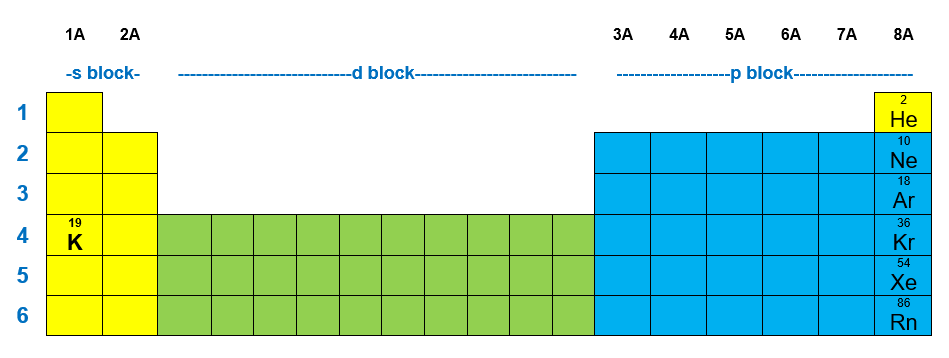# Problem: Each of the following elements is capable of forming an ion in chemical reactions.By referring to the periodic table, predict the charge of the most stable ion of K.

###### FREE Expert Solution

We have to determine the most stable ion of potassium (K).

We can start solving this problem by writing the electron configuration of potassium and the noble gas closest to it. We will write the electron configuration of K and Ar.• In a neutral atom:

Atomic number= # of protons = # of electrons

K: atomic number = 19 → 19 protons & 19 electrons

Ar: atomic number = 18 → 18 protons & 18 electrons

86% (440 ratings)###### Problem Details

Each of the following elements is capable of forming an ion in chemical reactions.

By referring to the periodic table, predict the charge of the most stable ion of K.

Frequently Asked Questions

What scientific concept do you need to know in order to solve this problem?

Our tutors have indicated that to solve this problem you will need to apply the Periodic Table: Charges concept. You can view video lessons to learn Periodic Table: Charges. Or if you need more Periodic Table: Charges practice, you can also practice Periodic Table: Charges practice problems.